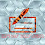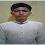-->

# Definition of Determinants

Determinants are functions with quadratic matrix variables and real values. In this article we begin the study of real value functions of a matrix variable, which is a function that associates a real number f (x) with an X matrix. Assessment of a function like that is called a determinant function.

The definition of determinant will be given inductively using minor and cofactor definitions. Utilizing the deteminan properties associated with elementary row operations, an easy way to calculate determinants is formed. On this occasion we will also discuss the application of determinants in solving a linear system using the Cramer rules.

Each square A matrix is ​​always associated with a scalar called deteminan the matrix, and we write it as det (A) or |A|. Before starting with a more general one, we first take a matrix of size (2 x s) as follows:

Defined:

Example 1

Note: We must distinguish parentheses for the matrix "[]" with the determinant "| | "
Before explaining definitive determinants, it is first discussed about permutations, inversions and their classifications, and the results of elements.

Definition of Permutation,
A permutation of a set of integers {1, 2, ..., n} is an arrangement of these integers according to a rule without omitting or repeating these numbers.

Example 2.
There are six different permutations of the set of integers {1, 2, 3}. These permutations are:
(1, 2, 3)          (2, 1, 3)          (3, 1, 2)
(1, 3, 2)          (2, 3, 1)          (3, 2, 1)

One method that is systematically and easily used to compile the results of permutations is to use a permutation tree.

Generally, the set (1, 2, ..., n) will have n (n-1) (n-2) ... 2.1) = different permutations. To declare a general permutation from the set {1, 2, .., n}, we will write (j1, j2, ..., jn). Here, j1 is the first integer in permutation, j2 is the second integer in the permutation and so on. An inverse is said to occur in a permutation (j1, j2, ..., jn) when a larger integer precedes a smaller integer.

Example 3.
Permutation (4  3  2   1)
(j1, j2, j3 , j4)

There are five inversions, namely:
1. j1 = 4 precedes j2 = 3, even though 3 <4
2. j1 = 4 precedes j3 = 1, whereas 1 <4
3. j1 = 4 precedes j4 = 2, even though 2 <4
4. j2 = 3 precedes j3 = 1, even though 1 <3
5. j2 = 3 precedes j4 = 2, even though 2 <3

Definition A permutation is called even if the total number of inverses is an even integer and is called odd if the inverse is an odd whole number.

Example 4.
The table below will classify various permutations {1, 2, 3} as even or odd.

 Permutation Number of Inversions Classification (1, 2, 3) 0 Even (1, 3, 2) 1 Odd (2, 1, 3) 1 Odd (2, 3, 1) 2 Even (3, 1, 2) 2 Even (3, 2, 1) 3 Odd

Review the matrix n x n

What we mean by the elementary multiplication result of A is every product of n entries from A, which cannot have two factors coming from the same line or from the same column.

Example 5.
Arrange all elementary multiplication results from matrix.

(i)   because the factor of every elementary multiplication has two factors, and because factors come from different rows, an elementary product can be written in the form of:
a1­­_ a2_
where blank points indicate column numbers. Since there are no two factors in the multiplication result coming from the same column, then the column must be 1 2 or 2 1. Then the elementary multiplication results are only a11a22 and a12a21

(i)    Because each elementary product has three factors, each of which comes from a different line, an elementary product can be written in form.
a1­­_ a2_a3_
because there are no two factors in the multiplication coming from the same column, then the column number has no repetition, as a consequence: the column numbers must form a permutation of the set {1, 2, 3}. Permutation 3! = 6 this produces the arrangement of elementary multiplication results below,

a11a22a33                a12a21a33        a13a21a32
a11a23a32              a12a23a31        a13a22a31

As shown by the example above, an A matrix of size n x n has n! Elemental multiplication results. The elementary multiplication results are multiplications in the form of a1j1a2j2...anjn, where (j1, j2, ..., jn) is a permutation of the set (1, 2, ..., n). What we mean by an elementary product multiplied by A is an elementary product of a1j1a2j2...anjn multiplied by +1 or -1. We use the "+ (plus)" if (j1, j2, ..., jn) is an even permutation and the "- (negative)" if (j1, j2, ..., jn) is an odd permutation.

Example 6.
Arrange all elementary multiplication results marked from matrix into a table.

(i)

 Elemental Multiplication Results Permutation associated Even or Odd Elemental Multiplication marked a11a22 (1, 2) Even a11a22 a12a21 (2, 1) Odd - a12a21

(ii)

 Elemental Multiplication Results Permutation associated Even or Odd Elemental Multiplication marked a11a22a33 (1, 2) Even a11a22a33 a11a23a32 (2, 1) Odd -a11a23a32 a12a21a33 (1, 2) Even -a12a21a33 a12a23a31 (2, 1) Odd a12a23a31 a13a21a32 (1, 2) Even a13a21a32 a13a22a31 (2, 1) Odd -a13a22a31

Let A be a quadratic matrix. The determinant of matrix A, expressed by det (A), is defined as the sum of all elementary multiplication results marked with A.

Example 7.

From the example above, to be able to more easily remember the formula of the results of elementary multiplication, then consider the one below,

Determinants are then calculated by summing the multiplication results in the arrows that point to the right and subtracting the arrows pointing to the left. So, we conclude that the determinant of A is often symbolically written as

Where indicates that the terms must be added to all permutations (j1, j2, ..., jn) and the symbols "+" or "-" can be selected in each tribe according to whether the permutation is even or odd.

Example 8.
Calculate the determinants of

Using the method above will get a value,

det(A)=3 x (-2) –(-1)x4=-10
det(B)=(1X5X9)+(2X6X7)+(3X(-4)X(-8))-(1X6X(-8)-(2X(-4)X9)-(3X5X7)
det(B)=45+84+96-105-(-48)-(-72)=240

The method as above, specifically for a 3 x 3 matrix is ​​called the SARRUS Method

Note:
The method above does not apply to determinants of 4 x 4 matrix or for higher matrix. Direct calculation of a 4 x 4 determinant will involve a calculation of 4! = 24 elementary multiplication results marked. Thus, the remaining part will be developed using determinant properties that will simplify the calculation.

### 2 Responses to "Definition of Determinants"

1.Mau seberapa ringannya matematika ini tetep aja paling ngablu aku hahaha

2.kayaknya si agan guru matematika nih, hhehe...mantap. selain bermanfaat ilmunya juga dapat dolar..hehe

saya paling gak mudeng matematika gan, apalagii pakai bhs inggris. wkwk

thx shering nya, salam bw

mediaweb4u